## Planar Graph

A Graph is planar if it can be drawn in a Plane without Edges crossing (i.e., it has Crossing Number 0). Only planar graphs have Duals. Ifis planar, thenhas Vertex Degree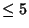. Complete Graphs are planar only for. The complete Bipartite Graph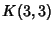in nonplanar. More generally, Kuratowski proved in 1930 that a graph is planar Iff it does not contain within it any graph which can be Contracted to the pentagonal graphor the hexagonal graph.can be decomposed into a union of two planar graphs, giving it a Depth'' of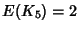. Simple Criteria for determining the depth of graphs are not known. Beineke and Harary (1964, 1965) have shown that if(mod 6), then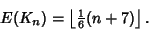The Depths of the graphsfor, 10, 22, 28, 34, and 40 are 1, 3, 4, 5, 6, and 7 (Meyer 1970).

See also Complete Graph, Fabry Imbedding, Integral Drawing, Planar Straight Line Graph

References

Beineke, L. W. and Harary, F. On the Thickness of the Complete Graph.'' Bull. Amer. Math. Soc. 70, 618-620, 1964.

Beineke, L. W. and Harary, F. The Thickness of the Complete Graph.'' Canad. J. Math. 17, 850-859, 1965.

Booth, K. S. and Lueker, G. S. Testing for the Consecutive Ones Property, Interval Graphs, and Graph Planarity using PQ-Tree Algorithms.'' J. Comput. System Sci. 13, 335-379, 1976.

Le Lionnais, F. Les nombres remarquables. Paris: Hermann, p. 56, 1983.

Meyer, J. L'épaisseur des graphes completeset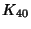.'' J. Comp. Th. 9, 1970.